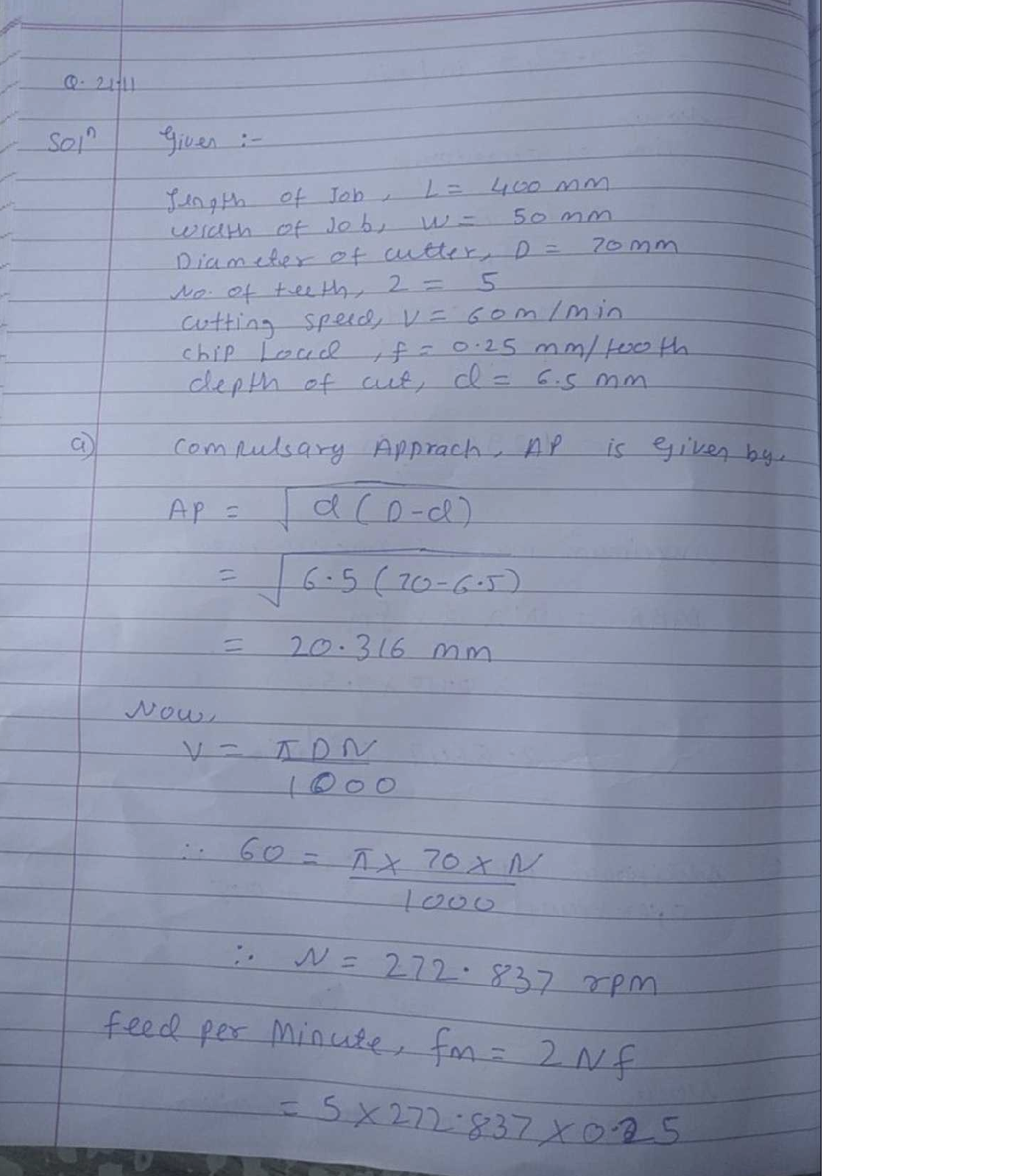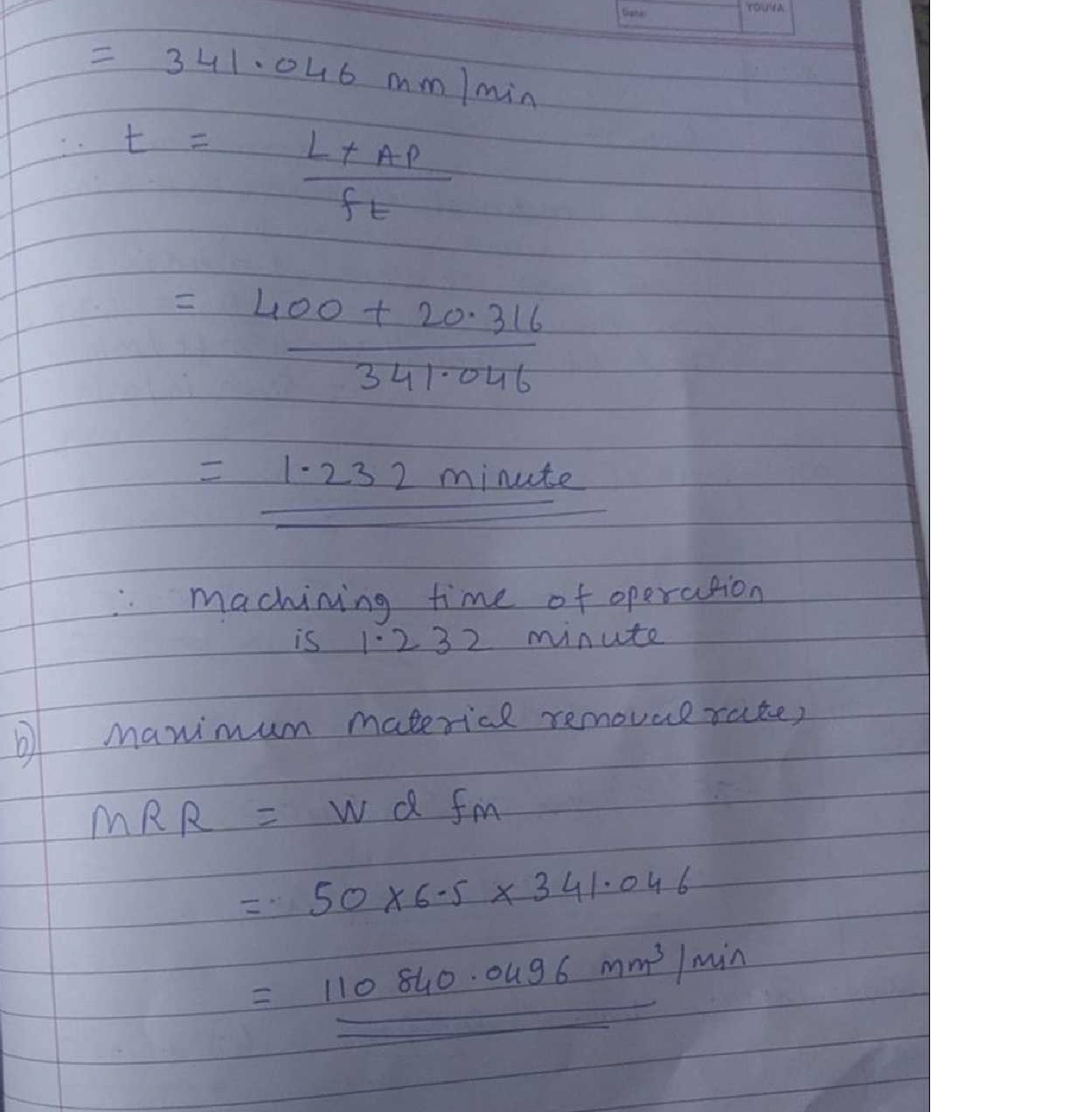Question

Manufacturing Process

(S) (SI units) Peripheral milling is performed on the top surface of a rectangular work part that is400 mm long by 50 mm wide. The milling cutter is 70 mm in diameter and has five teeth. It overhangs the width of the part on both sides. Cutting speed = 60 m/min, chip load = 0.25mm/tooth, and depth of cut = 6.5 mm. Determine (a) machining time of the operation and (b)maximum material removal rate during the cut.Verified### Question 42797Manufacturing Process

Problem #1: Part a in Figure 1** is to be produced using impression forging process.We are using a small billet/slug shown in Figure 2, as the input to the system. Assumingthe specific weight of the metal is =0.283 lb/in³ , answer the following questions
1)- is the input material satisfy the requirement of the impression forging (i.e., size of the input material)
3)-In case one: a)- Is the output of this process readily useful or need further processing.Explain why
b)- what is the yield of the system
4)- for a production rate of 500 units/day, determine material loss per month. Use the following assumption Day =8 hours, week =40 hours and a month = 4.2 weeks

### Question 42534Manufacturing Process

Problem 2: How much faster can you cook a potato in a convection oven versus a standard oven? Assume that the potato can be approximated as a cylinder with a diameter of 3 cm and length of 4 cm, with density p=950 kg/m^3, thermal conductivity k=0.8 W/m*K, and specific heat Cp=4.6 kJ/kg*K. The air temperature in both ovens is 190°C. and the potato starts at 20°C.
(a) Assuming a convection coefficient of h=8 W/m^2*K in a standard oven, is it appropriate to use a lumped capacitance approach? Calculate the time required to heat the potato to a temperature of 140°C.
(b) Assuming h=15 W/m^2*K in a convection oven, is the same approach still appropriate? Calculate the time required to heat to the same temperature.

### Question 42533Manufacturing Process

Problem 1: An interesting feature of some types of additive manufacturing is the ability to print functionally graded materials, where properties vary with direction. Consider a bar of length L=4 cm and heigh tH=1 cm, with a temperature of 20°C at x=0 and a temperature of 100°C atx=L. It is insulated (adiabatic) on its upper and lower faces.
(a) If the bar is constructed of a material with constant thermal conductivity k=115 W/m-K, calculate the magnitude of the heat transfer rate per unit depth (in W/m). Plot the temperature through the bar in the ex-direction from x=0 to L.
(b) Assume that another bar is constructed of a functionally graded material that starts at 20 W/m-K atx=0, and increases linearly to 400 W/m-K at x=L; that is, k(x)=[20+380*(x/L)] W/m-K, where x is in meters.Using the steady finite difference method with five nodes, determine the temperatures at each node and plot on the same figure as in part (a). Some important notes: i) assume each finite-difference control volume has a constant conductivity within it that corresponds to the x-location of that node; ii) when calculating the heat flux from the west face, use the k value from the west control volume, and when calculating east face heat flux, use the k value from the east control volume (this is not precisely correct but is reasonable). How does your plot differ from part (a)?
(c) Estimate the heat transfer rate per unit length through the bar at x=0 using temperatures at nodes 1and 2 and the information above. How does this compare to part (a)? Ans: -1694 W/m (or 1694 W/m in the negative x-direction)

### Question 41087Manufacturing Process

(b) Evaluate the resistance per unit length (R/L) for water flow (viscosity u= 1001.65x105) in micro channels that have three different shapes: (a) circular tube,(b) rectangular tube and (c) V grooves.

### Question 41083Manufacturing Process

(Working Mechanism: When you apply a high voltage, electrons emit from the micro tip to the bottom plate. The electron current (A) is proportional to the vacuum level of the Chamber. That way, if you calibrate the electron current with vacuum, you can measure the pressure of vacuum.This kind of sensors are good to measure high vacuum levels: 10*-10 Pa)
2. Consider the following electron emission type pressure sensor device. It is made by using wafer-bonding technology. The device is finalized by bonding two components (emitter membrane to the collecting plate). Give detailed processing steps of this device.

### Question 41052Manufacturing Process

a) Which casting process would you recommend for the manufacture of an internal combustion engine block and why? Explain briefly the process that you have selected.
b) Pure aluminium around 700°C is being poured into a sand casting mould. The metal level at the top of the sprue (pouring basin) is 32cm above the mould cavity. A circular runner connects the end of the sprue with the mould cavity.
i) Assuming that the velocity of liquid metal at the top of the sprue (pouring basin) is zero,what is the velocity of the metal at the bottom of the sprue?
ii) What runner diameter is needed to ensure aReynolds number of 2000?
You should assume constant pressure in the sprue and no significant friction. Pure aluminum has a density of 2700 kg/m3 and a viscosity of 0.0015 Ns/m? around 700°C.
\text { Bernoulli equation } \frac{u^{2}}{2 g}+\frac{p}{g \rho}+h=\text { constant }
\text { Reynolds number Re }=\frac{\rho u D}{\mu}
c) Explain with the aid of a schematic the electroplating process of nickel onto copper.What are the benefits of coating copper objects with nickel?

### Question 41051Manufacturing Process

a) i) Explain the difference between cold and hot working in metals. ii) Define the re-crystallization temperature. iii) Explain why the material strength increases during cold working.
b) Explain the effects of the three stages of annealing i.e. recovery, recrystallisation and grain grown on the structure and properties of cold worked metal.
c) During a tensile test, a metal has a true strain of 0.08 at a true stress of 2.32x108 Pa.Later, at a true stress of 3.91x108 Pa, the true strain is 0.22. Determine the flow curve parameters n and K.
The relationship between true stress and true strain in the plastic region can be expressed as:
\sigma_{\mathrm{T}}=K \varepsilon_{T}^{n}
\text { where } \sigma_{T} \text { is the true stress and } \mathrm{ET} \text { is the true strain. }

### Question 41041Manufacturing Process

18.15. (USCS units) A cylindrical work part with diameter 2.5 in, and height = 2,5 in is upset forged in an open die to a height -1.5 in. Coefficient of friction at the die-work interface = 0.10. The work metal has a strength coefficient - 40,000 lb/in' and strain hardening exponent - 0.15. Yield strength-15,750 lb/in',Determine the instantaneous force in the operation (a) just as the yield point is reached (yield at strain -0.002), (b) at height h- 2.3 in, (c) h= 2.1 in,

### Question 41040Manufacturing Process

18.10. (USCS units) A 4-in-thick aluminum slab is 10 in wide and 100 in long. It is reduced in one pass in a two-high rolling mill to a thickness - 3.5 in. The roll rotates at a speed - 12 rev/min and has a radius - 20in. The aluminum has a strength coefficient 25,000 lb/in' and a strain hardening exponent- 0.20 (Table3.4), Determine (a) roll force, (b) roll torque, and (e) power required to accomplish this operation.

### Question 41039Manufacturing Process

18.3 (Sl units) A series of cold rolling operations are to be used to reduce the thickness of a metal plate from 50 mm to 20 mm in a reversing two-high mill. Roll diameter - 600 mm, and coefficient of friction between rolls and work = 0.15. The specification is that the draft is to be equal on each pass. Determine (a)minimum number of passes required, and (b) draft for each pass?

### Submit query